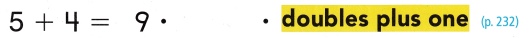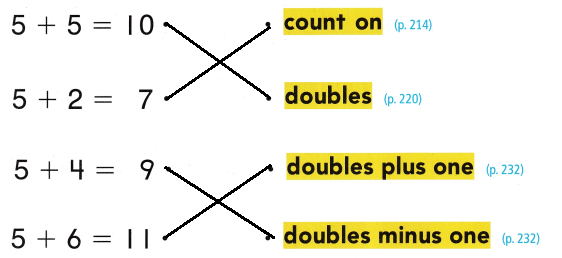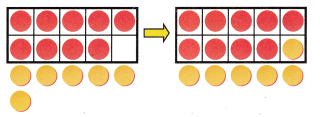Refer to our Texas Go Math Grade 1 Answer Key Pdf to score good marks in the exams. Test yourself by practicing the problems from Texas Go Math Grade 1 Module 6 Assessment Answer Key.

Vocabulary

Match each fact to a strategy you would use to solve.

Question 1.Is doubles
5 + 5 = 10

Question 2.count on
5 then 6 , 7

Question 3.Double minus 1
5 +5 = 10
10 – 1 = 9

Question 4.Double plus 1
5 + 5 = 10
10 + 1 = 11Explanation:
Matched each fact according to the strategy.

Concepts and Skills
Useand a ten frame. Show both addends. Draw to make a ten. Then write the new fact. Add. TEKS 1.3.D

Question 5.Explanation:
9 + 5
Breaking 5 as 1 and 4
9 + 1 = 10
10 + 4 = 14
9 + 5 = 14

Write to show how you make a ten.

Question 6.
What is 9 + 5?Explanation:
9 + 5
Breaking 5 as 1 and 4
9 + 1 = 10
10 + 4 = 14
9 + 5 = 14

Texas Test Prep

Question 7.
Which number sentence does this model show? TEKS 1.3.D(A) 10 – 9 = 1
(B) 10 + 9 = 19
(C) 10 + 8 = 18
Explanation:
10 + 8 = 18
It is a breaking ten model

Question 8.
Which number sentence matches the model? TEKS 1.3.D(A) 8 + 3 = 11
(B) 8 + 4 = 12
(C) 12 – 3 = 9
Explanation:
The number in box is 8
then 3 books
8 + 3 = 11

Question 9.
What is the sum of the doubles minus one fact? TEKS 1.3.D(A) 14
(B) 13
(C) 154 + 4 = 8
8 – 1 = 7
Double minus 1 fact

Question 10.
Which way shows how to make a ten to Solve 9 + 6? TEKS 1.3.D(A) 9 + 1 + 5
(B) 9 + 1 + 6
(C) 6 + 5 + 5